The idea behind the dot plot is to stack dots in columns to represent the distribution of the sample. In a way, a dot plot is very similar to a histogram as it plots counts vs. values. There are two rules to draw a dot plot:

• the diameter/width of the dots is equal to the bin width,
• each dot represents a single observation in the sample.

Let’s take a simple example to see how to build a dot plot with ggplot().

# ID
ID <- 1:100
# sample data
data <- rnorm(100, mean=30, sd=5)
# dataframe
df <- data.frame(ID,data)
str(df)
## 'data.frame':    100 obs. of  2 variables:
##  $ID : int 1 2 3 4 5 6 7 8 9 10 ... ##$ data: num  30.3 22.3 20.1 30.5 35.1 ...

Let’s map the data from the variable data by typing ggplot(my.dataframe, aes(data)) and use geom_dotplot() with a bin width of 1:

ggplot(df, aes(data)) +
geom_dotplot(binwidth = 1)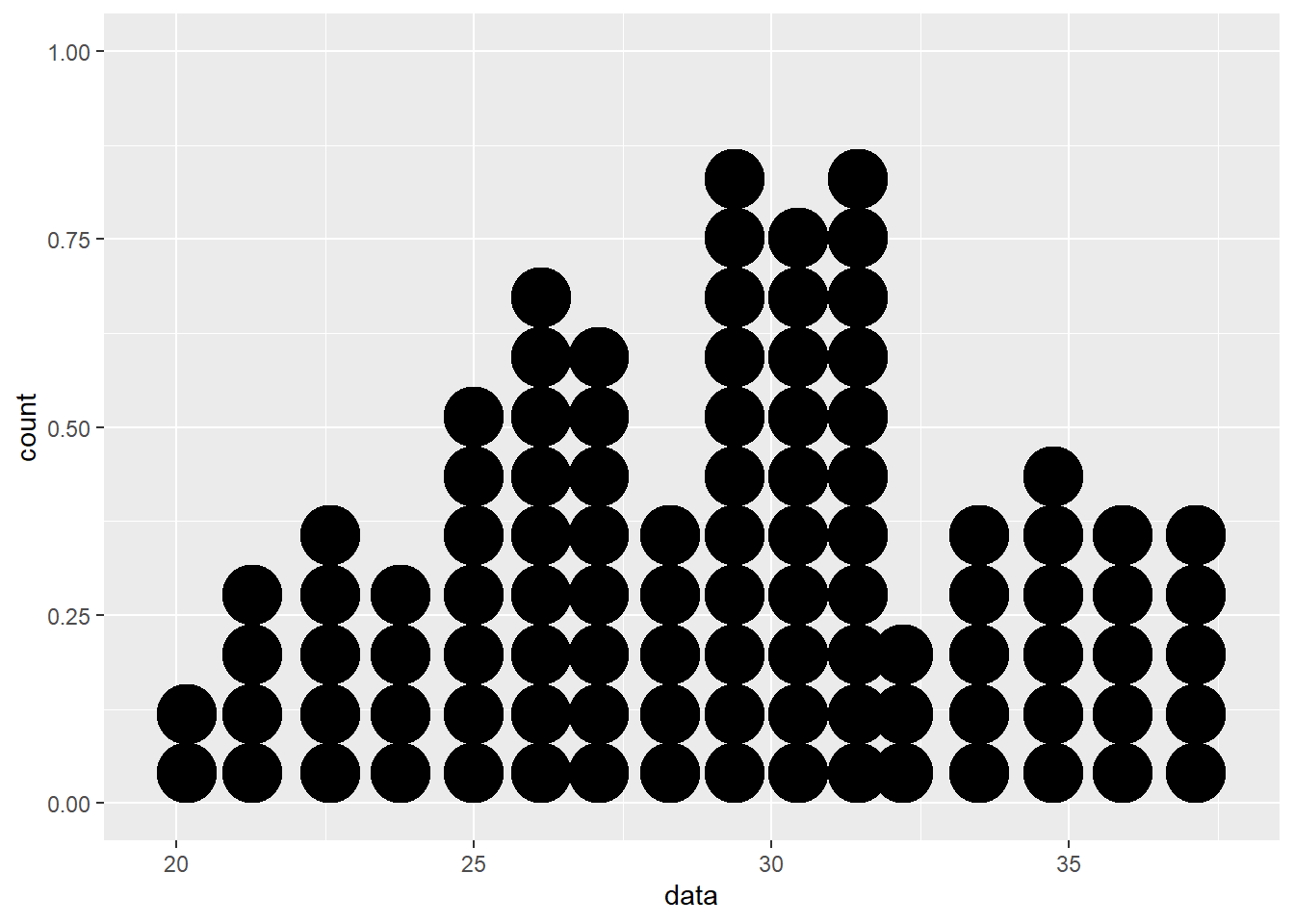As you may see here, geom_dotplot() creates a plot displaying counts on the Y-axis and the values of the variable data on the X-axis, in the same manner as histograms do. To highlight the similarities between dotplot and histogram, we can place the two plots drawn from the same data set next to each other:

ggplot(df, aes(data)) +             # histogram
geom_histogram(binwidth = 1)
ggplot(df, aes(data)) +             # dotplot
geom_dotplot(binwidth = 1)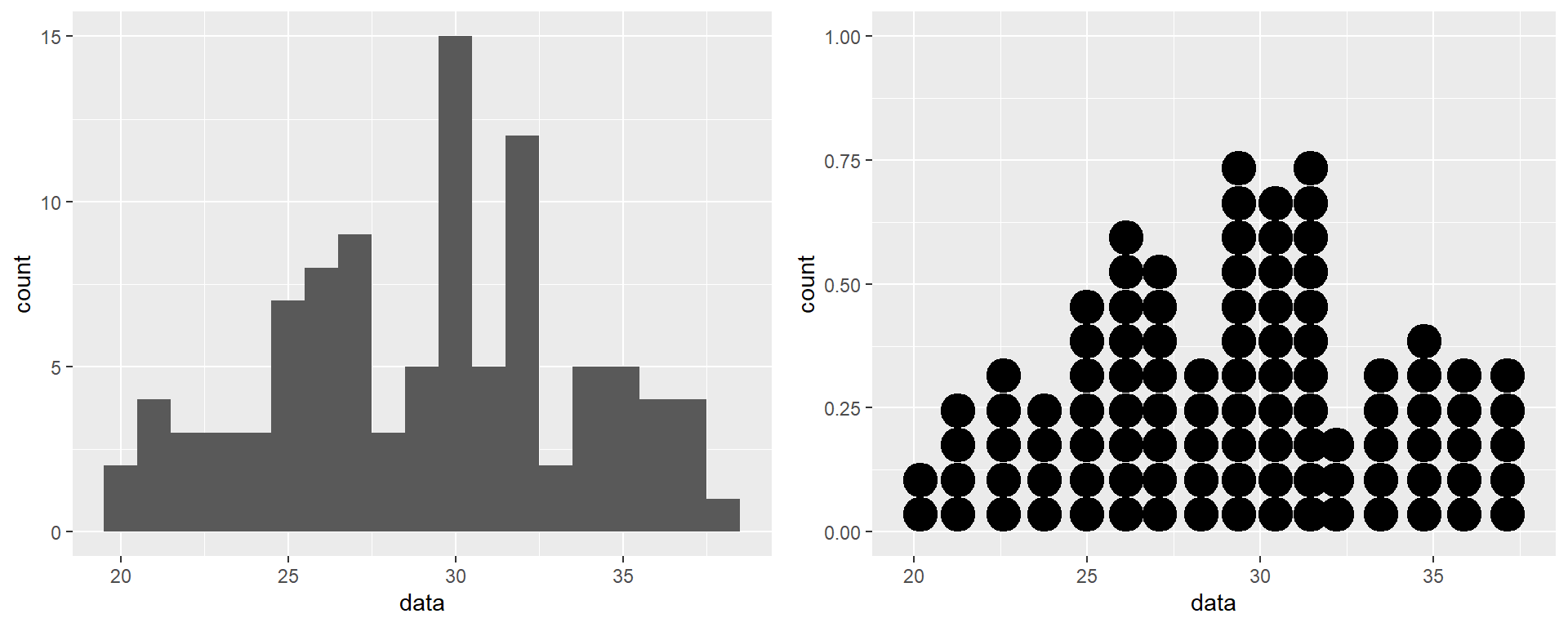Even though the shapes of these two plots are not strictly identical, they show similar patterns of distributions.

Using the argument binwidth=, it is possible to change the aspect of the dotplot. Changing the binwidth indeed changes automatically the diameter of the dots. However, this is quite tricky when you handle large data sets as the stacks of dots become very high when the diameter of the dots increases; the column go higher than the vertical limits of the chart, making it unreadable. Here is an example with binwidth=2 illustrating this issue:

ggplot(df, aes(data)) +
geom_dotplot(binwidth = 2)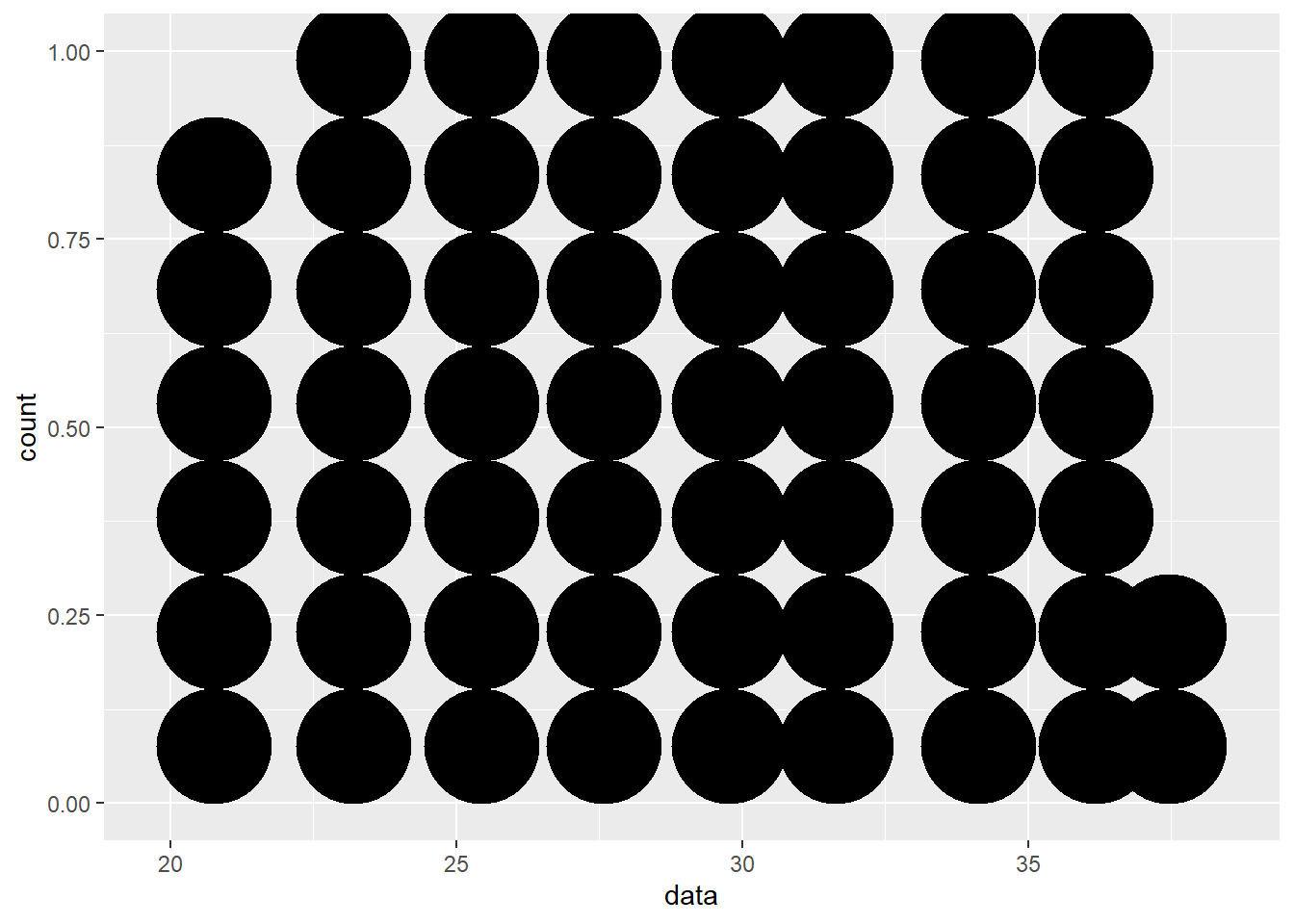Using stackdir="center", you can ask ggplot to stack the dots from the center, thus creating a symmetrical figure:

ggplot(df, aes(data)) +
geom_dotplot(binwidth = 1, stackdir = "center")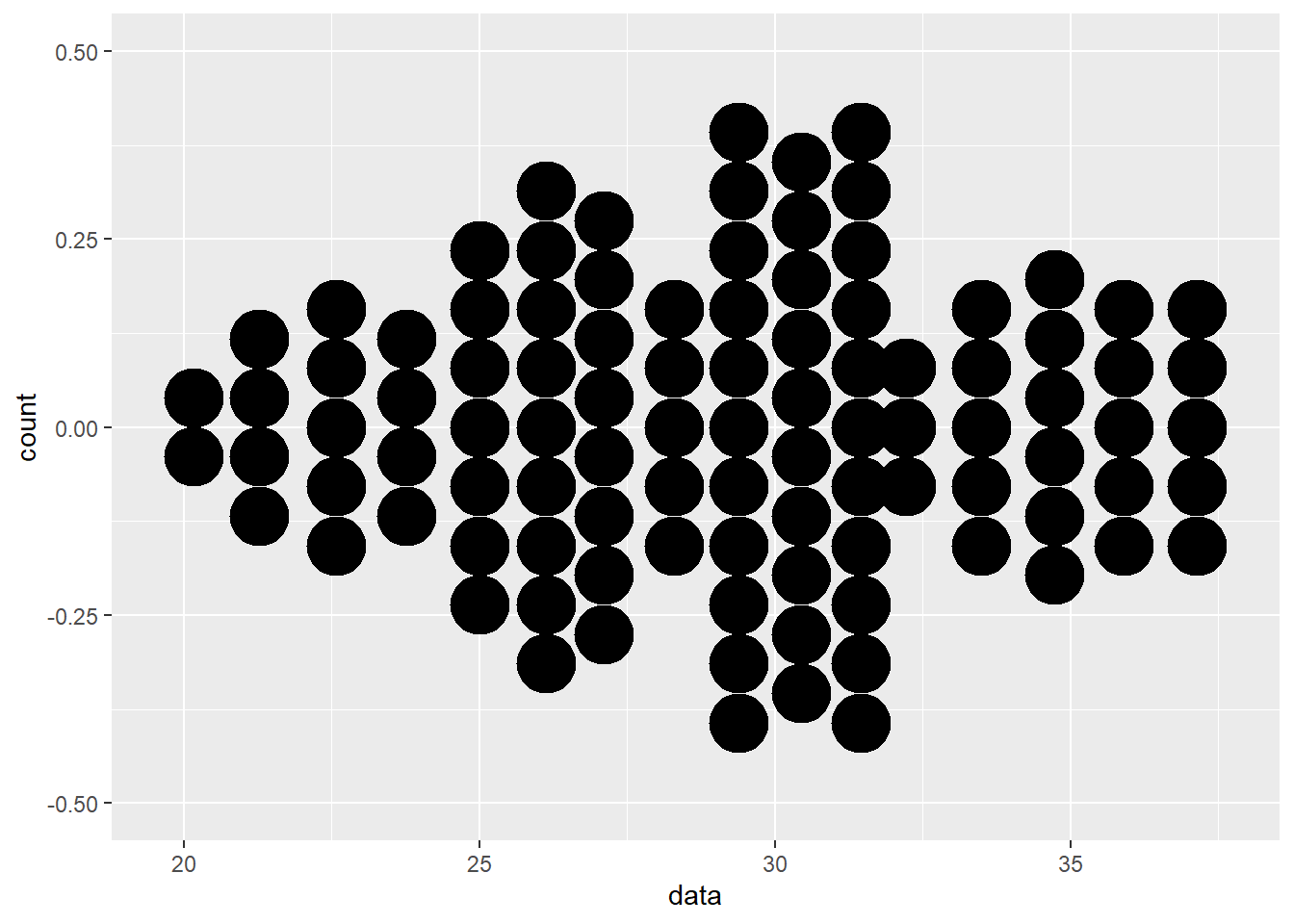And you can even plot the dots along the Y-axis, thus flipping the plot 90 degrees counterclockwise by using binaxis = "y".

ggplot(df, aes(x = 1, data)) +
geom_dotplot(binwidth = 1, stackdir = "center", binaxis = "y")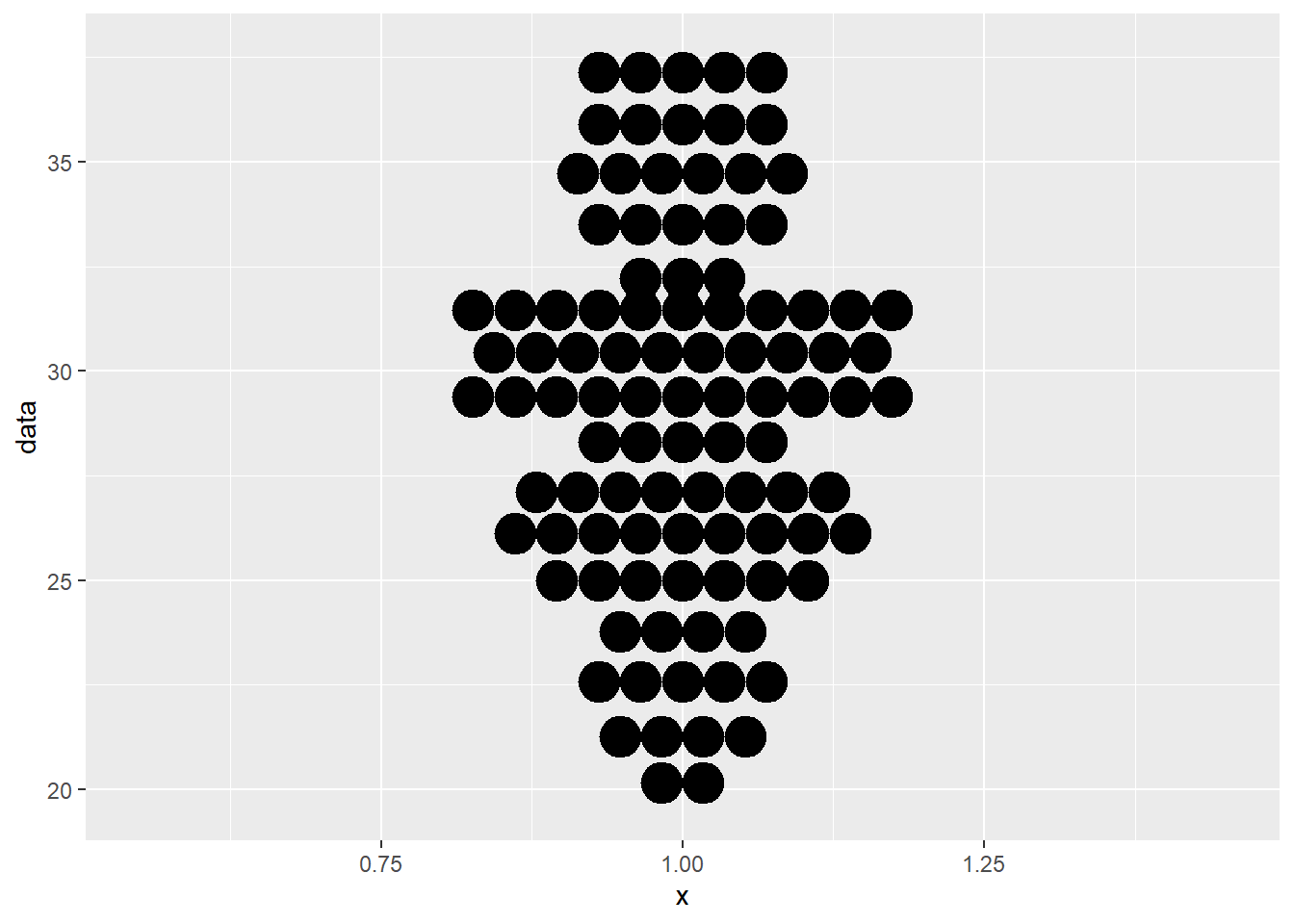Note that flipping the plot in this way requires that we map a new x variable in aes(). In our case, we used x = 1.

### Multiple dot plot

Until now, we have been drawing dot plots representing only one sample. We may use dotplots to compare several groups or samples by the means of fill=. To illustrate this, we need a more appropriate dataframe:

# ID
ID <- 1:99
# sample data
group <- rep(c("A","B","C"), each =33)
values <- c(runif(33, min=5, max=26), runif(33, min=25, max=36), runif(33, min=25, max=33))
# dataframe
df2 <- data.frame(ID, group, values)

Here is the code that creates this multiple dot plot, where x= refers to the variable group:

ggplot(df2, aes(x = group, y = values)) +
geom_dotplot(binwidth = 1, stackdir = "center", binaxis= "y")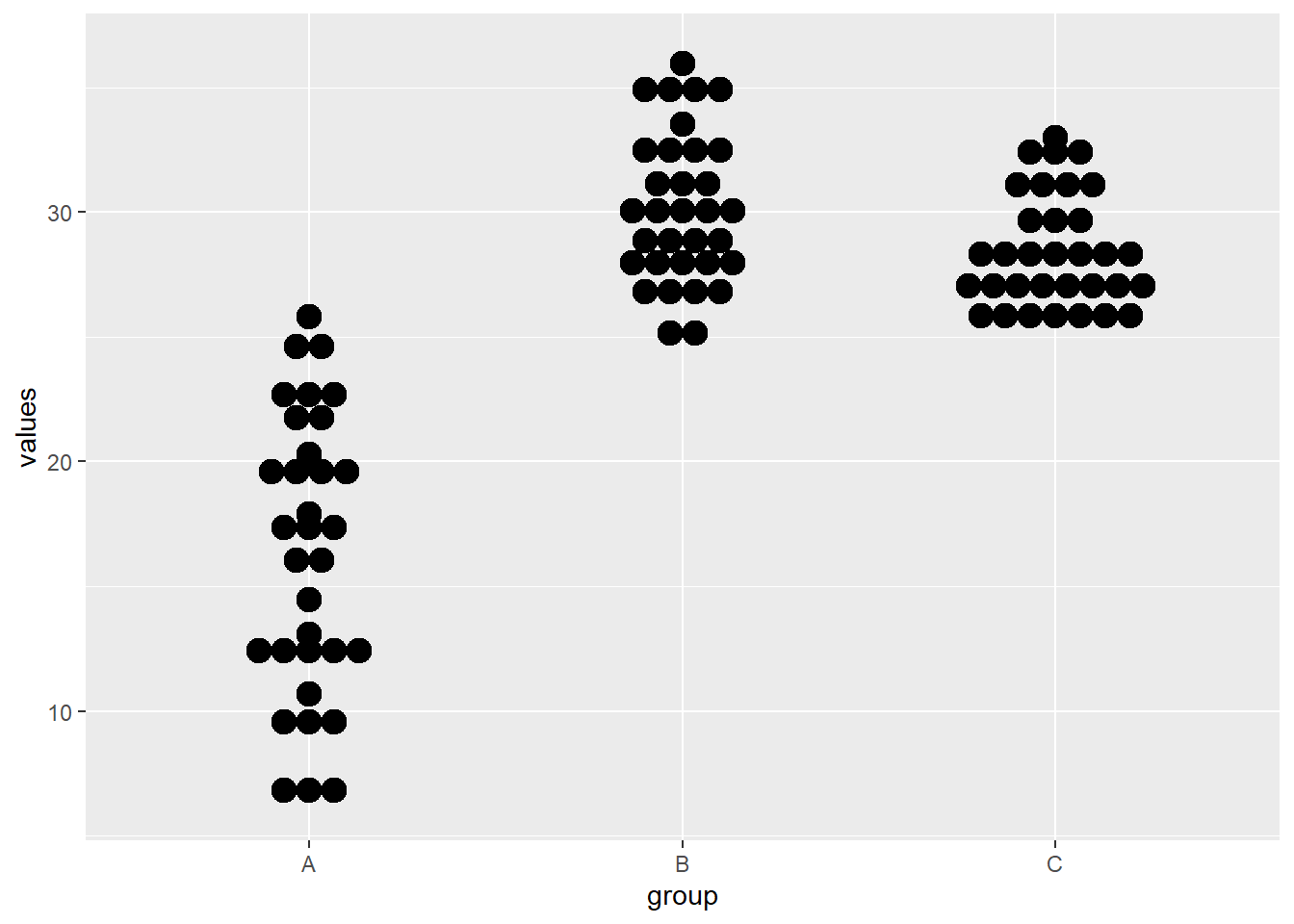And here is the same dot plot, this time with colors thanks to fill= and the default color palette in ggplot:

ggplot(df2, aes(x = group, y = values, fill = group)) +
geom_dotplot(binwidth = 1, stackdir = "center", binaxis= "y")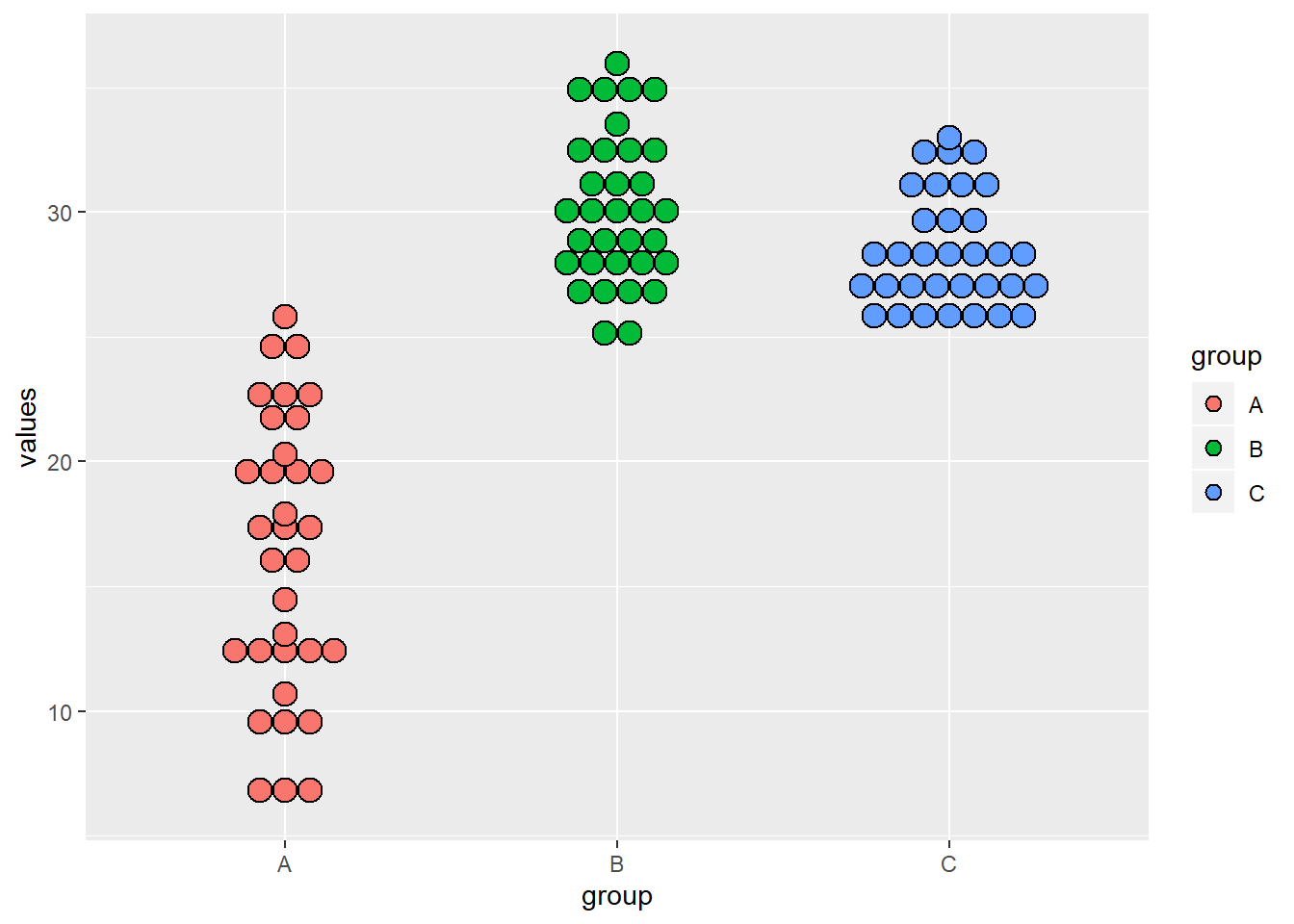Read this page to learn more about color palettes.

### Adding plot title, axis titles, ticks, labels and other essential elements

In this section, you will learn how to set/modify all the necessary elements that make a plot complete and comprehensible. Such elements are: